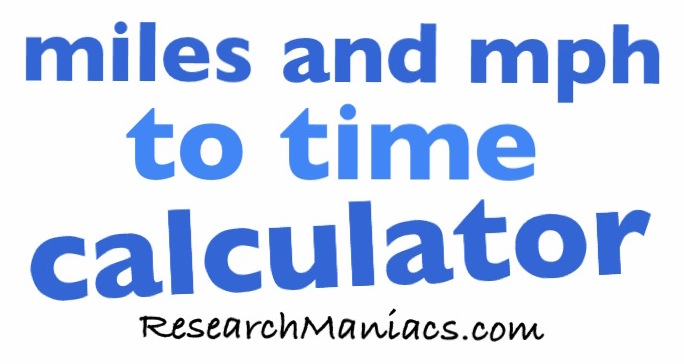How long does it take to drive 100 miles at 50 mph?The time it takes to drive 100 miles at 50 miles per hour (mph) is displayed below in hours, minutes, and seconds:

2 hours
0 minutes
0 seconds

Do you want to know how to calculate how long it takes to drive 100 miles at 50 mph? No problem. Here is how we did it:

1) Find the number of hours by dividing the distance by mph. The number of hours will be to the left of the decimal point:

100 miles / 50 mph
= 2.0
= 2 hours

2) Find the number of minutes by multiplying what is remaining from step 1 by 60 minutes. The minutes will be to the left of the decimal point:

0 x 60
= 0.0
= 0 minutes

3) Find the number of seconds by multiplying what is remaining from step 2 by 60 seconds. The seconds will be to the left of the decimal point:

0 x 60
= 0.0
= 0 seconds

Miles and mph to Time Calculator
Enter another distance (miles) and how fast you are going (mph) below and press "Time to Drive."

How long does it take to drive   miles at   miles per hour (mph)?

How long does it take to drive 100 miles at 51 mph?
Here is the next number of miles and mph we converted to time.